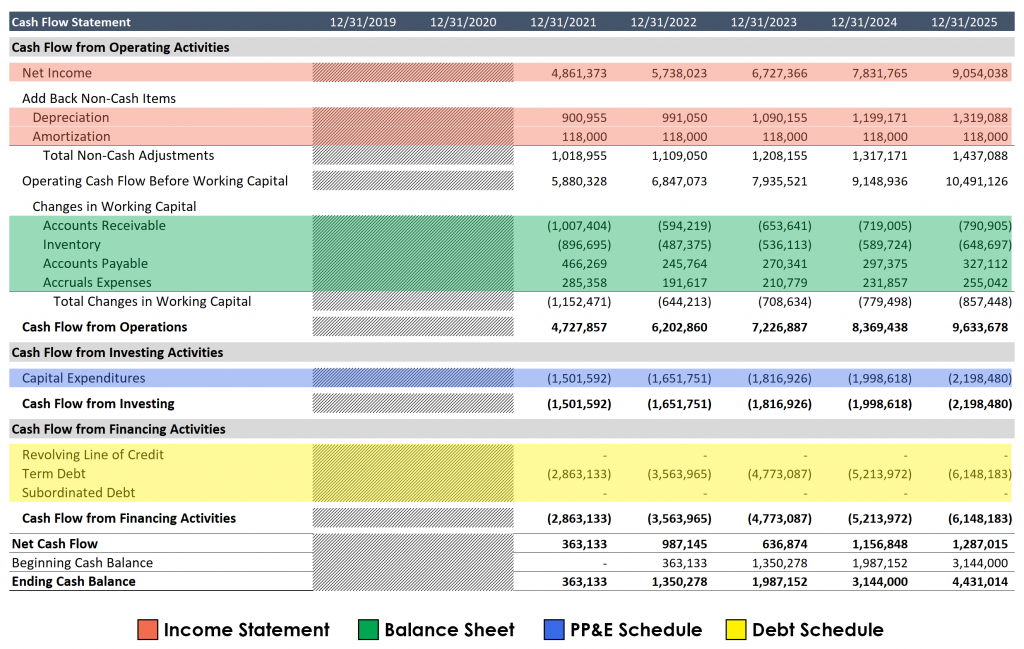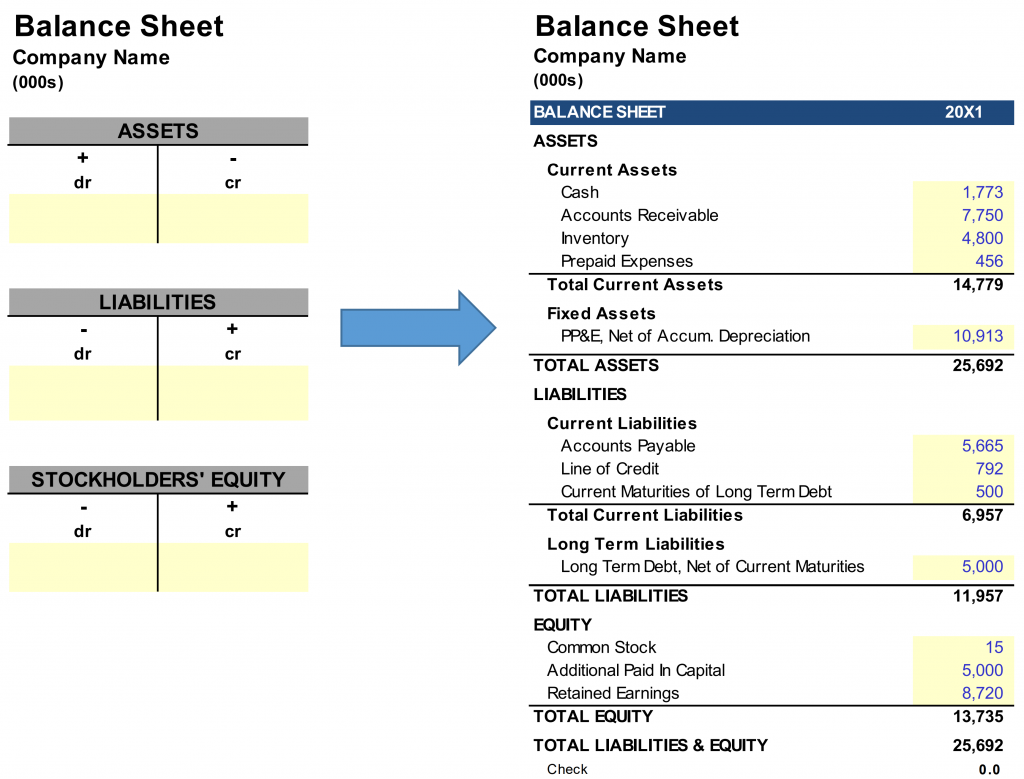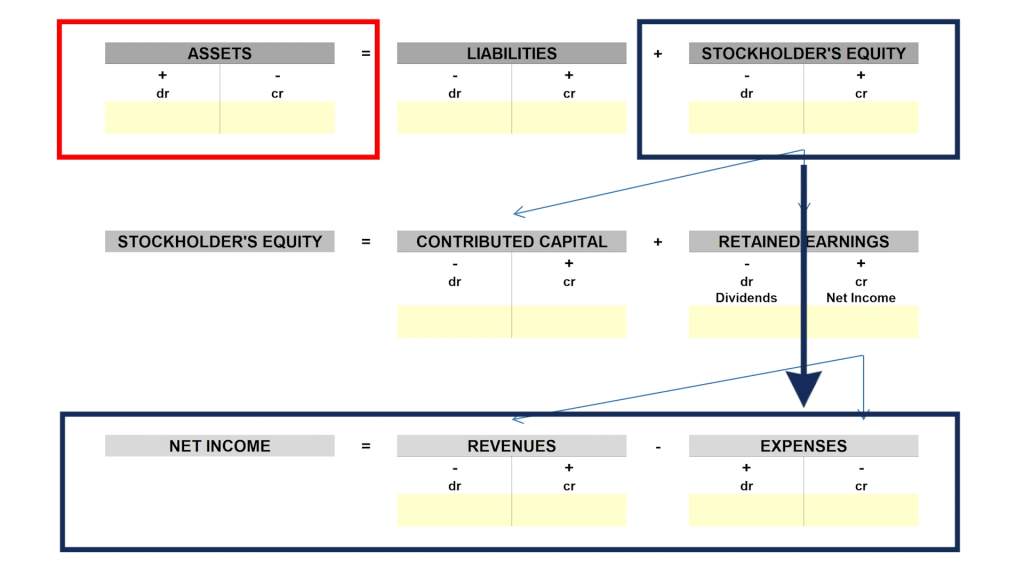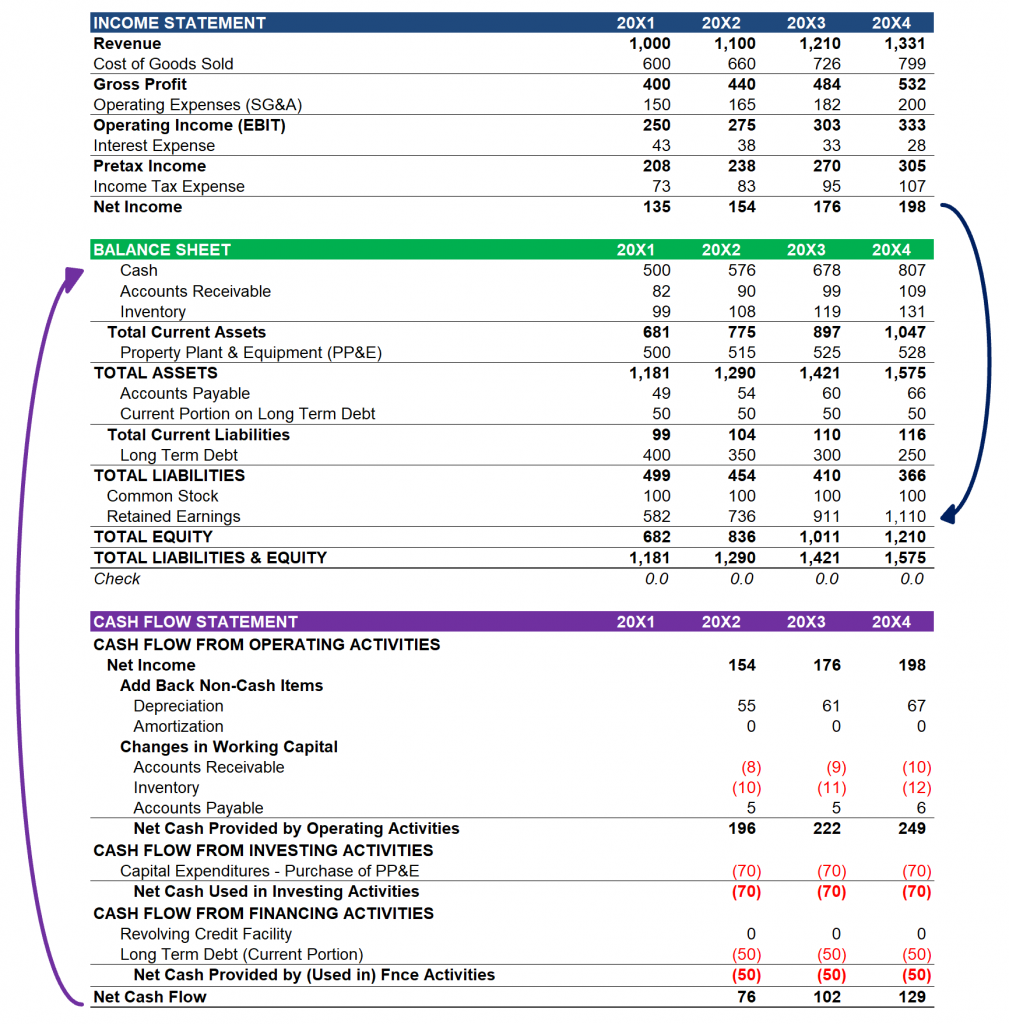Select Page

# How a Balance Sheet Balances

• Peter Lynch

An unbalanced balance sheet in a three-statement financial model can be a nightmare if you don’t understand the mechanics that would otherwise result in a balanced balance sheet. In this post we will explore how the cash flow statement balances a three-statement model, and we will include common errors that result in a broken model together with instructions on how to fix them.

The beautiful thing about accounting and the three-statement models it helps inform is that they create a closed system. What affects the income statement also affects the balance sheet, and any change on the balance sheet must be captured by the cash flow statement. As evidence of these deeply intertwined relationships, look no further than the fact that, at this point in the model building process, each line item on the cash flow statement has already been addressed on the income statement, balance sheet and the associated supporting schedules, with the exception of cash. This is visible in the image that follows.In a financial model, the purpose of the cash flow statement is to calculate the amount of cash generated in each accounting period, building from net income. The result, cash generated in the period, is added to the previous period’s ending cash balance to arrive at the cash balance for the period, which links back to the balance sheet. This sum is what balances the model. To understand why, let’s quickly review a concept first introduced in the Introduction to Financial Statements video series.

Recall that the balance sheet is just a formal presentation of the fundamental accounting equation (the assets of a company = its liabilities + its equity). If the accounting equation is rearranged, per the image below, you can see this relationship more clearly.If the cash flow statement adjusts the left-hand side of the equation (assets) by the company’s cash flow in that period, and the income statement adjusts the last part of the right-hand side of the equation (stockholders’ equity) by net income, then it follows that the cash flow statement, which starts with net income and ends with cash flow in period, is making adjustments so that the accounting equation holds true.  And that is how the accounting equation, and by extension the balance sheet, is balanced in financial models.To make this concept really stick, lets look at how this works using a financial model as the visual. Here you can see the link from the income statement to the balance sheet, where retaining earnings grows by the amount of net income in each accounting period. And then the cash flow statement adjusts net income for non-cash items and every change on the balance sheet to arrive at cash generated in the period. And this cash is added to the cash balance on the balance sheet.Mechanically, this is the most important concept to focus on as it relates to projecting the cash flow statement, because there is nothing worse than an unbalanced model. I cannot tell you how many times an unbalanced model kept me in my cubicle residence overnight. Enough that I created the Handbook for Analysts Screaming at their Monitors at 4 AM but later renamed it Balancing the Model. This link will walk you through the most common errors found in an unbalanced balance sheet and explain how to fix them as quickly as possible. Hopefully the framework provided in this post coupled with the tips available in Balancing the Model will keep you from punching a monitor 🙂